# 15 Multiple Choice Calculus

1.

Which of the following integrals represents the area of the region bounded by x = e and the functions f(x) = ln(x) and g(x) = log1/e(x)? (4 points)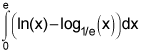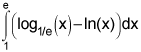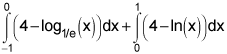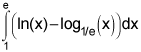2.

Which integral gives the area of the region in the first quadrant bounded by the axes, y = ex, x = ey, and the line x = 4? (4 points)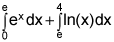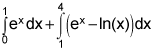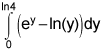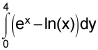3.

Find the area of the region bounded by the graphs of y = x2 – 4x and y = x – 4. (4 points)

 -4.500 4.500 2.333 None of these

4.

Find the area of the region bounded by the graphs of y = x, y = 4 – 3x, and x = 0. (4 points)

 0.375 2 4 None of these

5.

Find the number a such that the line x = a divides the region bounded by the curves x = y2 − 1 and the y-axis into 2 regions with equal area. Give your answer correct to 3 decimal places. (4 points)

1.

Use your calculator to find the approximate volume in cubic units of the solid created when the region under the curve y = cos(x) on the interval [0,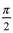] is rotated around the x-axis. (4 points)

 1 0.785 2.467 3.142

2.

Find the volume of the solid formed by revolving the region bounded by the graphs of y = x3, x = 2, and y = 1 about the y-axis. (4 points)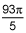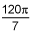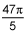None of these

3.

Which of the following integrals correctly computes the volume formed when the region bounded by the curves x2 + y2 = 25, x = 4 and y = 0 is rotated around the y-axis? (4 points)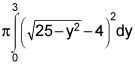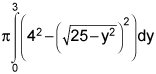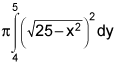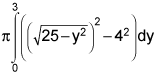4.

The base of a solid in the first quadrant of the xy plane is a right triangle bounded by the coordinate axes and the line x + y = 6. Cross sections of the solid perpendicular to the base are squares. What is the volume, in cubic units, of the solid? (4 points)

 36 18 108 72

5.

The base of a solid in the region bounded by the two parabolas y2 = 8x and x2 = 8y. Cross sections of the solid perpendicular to the x-axis are semicircles. What is the volume, in cubic units, of the solid? (4 points)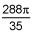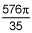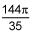8π

1.

Find the average value of f(x)=e2x over the interval [2, 4]. (4 points)

 1463.18 731.59 1517.78 23.6

2.

Find the velocity, v(t), for an object moving along the x-axis if the acceleration, a(t), is a(t) = cos(t) – sin(t) and v(0) = 3. (4 points)

 v(t) = sin(t) + cos(t) + 3 v(t) = sin(t) + cos(t) + 2 v(t) = sin(t) – cos(t) + 3 v(t) = sin(t) – cos(t) + 4

3.

Find the distance, in feet, a particle travels in its first 2 seconds of travel, if it moves according to the velocity equation v(t)= 6t2 – 18t + 12 (in feet/sec). (4 points)

 4 5 6 -1

4.

For an object whose velocity in ft/sec is given by v(t) = -3t2 + 5, what is its displacement, in feet, on the interval t = 0 to t = 2 secs? (4 points)

 6.607 2 -2.303 2.303

5.

A pitcher throws a baseball straight into the air with a velocity of 72 feet/sec. If acceleration due to gravity is -32 ft/sec2, how many seconds after it leaves the pitcher’s hand will it take the ball to reach its highest point? Assume the position at time t = 0 is 0 feet. (4 points)

 2.25 2.5 4.25 4.5